#### R – create scatterplot with ggplot2

R has pretty amazing capabilities for creating charts and graphs. One of the most common packages for this is ggplot2. However, it’s not the most intuitive package I have used in R. So, I figured I’d illustrate how to make some relatively simple scatterplots in R using ggplot2. I’ll likely post instructions on how to create other graphs/charts in ggplot2 as well.

As with most of my R examples, I’m going to use the 2010 wave of the General Social Survey (R version here) to illustrate. You can open that file in R and follow along.

The point of a scatterplot is to visually examine the relationship between two variables. Since it’s well-known that there is a relationship between how much education someone has and how much money they make, let’s examine that relationship visually. The two variables in the GSS we’ll use are: EDUC (years of education ranging from 0 to 20, with 12 being a high school diploma and 16 a Bachelor’s degree) and REALRINC (the respondent’s income in 1986 dollars). Just because I want the resulting scatterplots to be more accurate, let’s go ahead and adjust the REALRINC into 2010 dollars first. Per this website, we need to multiply each income listed in the 2010 GSS by 1.989 to get 2010 dollars from 1986 dollars:

`GSS2010\$REALRINC2010 <- GSS2010\$REALRINC * 1.989`

Let’s just make sure the values make sense. To do that, first pull the first 10 incomes:

`head(GSS2010\$REALRINC, 10)`

The “head” command tells R to simply list the first “10” values in the variable “REALRINC” and print them in the console. Here’s what I got as output:

 42735.0 3885.0 NA NA NA 5827.5 42735.0 NA NA NA

We can check to see what the corresponding value in 2010 dollars is for the first value, \$42,735, with the following command:

`GSS2010\$REALRINC2010[match(42735,GSS2010\$REALRINC)];`

The value I got was \$84,999.92, which is \$42,735 * 1.989. Groovy. Our formula worked.

Now to create the scatterplot. Install ggplot2 (I’m using version 3.2.1) and load the library.

`install.packages("ggplot2")library(ggplot2)`

Now begins the rather bizarre part of working with ggplot2 – building the commands to create the charts.

The basic code for creating plots in ggplot2 is odd. You create a base object that includes the variables that will be used for creating the chart like this:

`scatterEDUCxINCOME <- ggplot(GSS2010, aes(EDUC, REALRINC2010))`

The first portion, “scatterEDUCxINCOME” is the base object.

ggplot” calls the ggplot2 package.

In the parentheses is, first, the database (GSS2010), followed by the “aesthetic” components of the chart (“aes” stands for aesthetic). In this case, it’s the two variables we’re going to use: EDUC and REALRINC2010.

This base object is really just the data for the scatterplot but not the scatterplot itself. To illustrate, you can tell R to display the base object:

`scatterEDUCxINCOME`

You’ll get some of the pieces of a scatterplot, but not the points:

To overlay the scatterplot onto the base object, you have to tell R what objects you want to be overlaid. Obviously, that should include the points, which would be done like this:

`scatterEDUCxINCOME + geom_point()`

geom_point()” tells R to overlay the geometric points for the scatterplot onto the base layer. The parentheses are useful as they allow for additional commands or modifiers for the points, as I’ll illustrate below.

Two points to note with the code above. First, when you run it, it will generate the scatterplot (see image below).

Second, you can actually save this entire piece of code into the base object, like this:

`scatterEDUCxINCOME <- scatterEDUCxINCOME + geom_point()`

However, doing so converts your base object into the scatterplot with the geometric points included. Since you may want to modify your scatterplot with additional features, it’s generally a good idea to not do that. Keep your base object simple and overlay things on top of it as you go until you get to where you want to be.

As noted, the geom_point() code can be modified. One of my favorite modifications is to add a command that adds a little bit of entropy to the scatterpoints, which is helpful when you have a large dataset. Otherwise, all the points are basically stacked on top of each other. Here’s how:

`scatterEDUCxINCOME + geom_point(position = "jitter")`

Of course, a scatterplot should have labels – a title and axis labels. ggplot2 tries to add the axis labels based on the names of the variables, but you can overlay these as objects onto the base object as well, like this:

`scatterEDUCxINCOME + geom_point() + ggtitle("Scatterplot of Educational Attainment and Individual Income") + labs(x = "Educational Attainment (in years)", y = "Individual Income")`

ggtitle adds a title to the chart.

labs(x = “”, y = “”) adds the labels for the x and y axes with the labels inside the quotes.

Two additional features are helpful in a basic scatterplot. First, adding a line to the chart to illustrate the relationship is helpful. I’ll show two. First, a standard regression line is always nice:

`scatterEDUCxINCOME + geom_point() + geom_smooth(method = "lm", color = "red", alpha = 0.2, fill = "blue") + ggtitle("Scatterplot of Educational Attainment and Individual Income") + labs(x = "Educational Attainment (in years)", y = "Individual Income")`

The code to add a smoothing line is geom_smooth. In this case, I modified that line to make it linear (“lm”), make it red (color = “red”), and changed the fill of the error around the line (fill = “blue”). The last modification was to change the transparency of the fill for the error (alpha = 0.2).

Other smoothing options are available. The default simply runs a best fitting line through the scatterplot:

`scatterEDUCxINCOME + geom_point() + geom_smooth() + ggtitle("Scatterplot of Educational Attainment and Individual Income") + labs(x = "Educational Attainment (in years)", y = "Individual Income")`

Finally, the scatterpoints can be modified in a variety of ways (e.g., thickness, transparency, color, etc.). Here’s a simple illustration:

`scatterEDUCxINCOME + geom_point(position = "jitter", size = 4, colour = "green")`

The points options that can be modified are detailed here.

A script file with the above commands is available here.

NOTE: This was done in R version 3.5.2.

2,133 total views,  1 views today

#### R – find cases (rows) that match specific criteria

I regularly need to find a specific case or set of cases that meet some criteria when analyzing data, often so I can modify those values for one reason or another. The easiest way I have found to find such values in R is the “which” function.

As with most of my R examples, I’m going to use the 2010 wave of the General Social Survey (R version here) to illustrate. You can open that file in R and follow along.

In the 2010 GSS there is a variable for race (RACE). The options are: 1 = WHITE, 2 = BLACK, 3 = OTHER. To find all of the cases with a “3” in the dataset, I would use the following code:

``which(GSS2010\$RACE == "3", arr.ind = TRUE)``

Here’s what the command is doing…

which” is the function that tells R to search for information that meets the criteria detailed in the parentheses.

GSS2010 is the name of the dataset.

RACE is the name of the variable in the dataset. By including the name of the variable, we restrict R to searching just inside that variable rather than the whole dataset. (The \$ tells R that RACE is a variable inside the GSS2010 dataset.

The “==” indicates “equals” in R.

The target value, which can be text, characters, or numbers, goes inside the quotes. In this case, we wanted to find all of the cases with the number “3” which is code for “OTHER.”

arr.ind = TRUE tells R to include the index if the result is an array.

In the 2010 GSS, if you type in the code above, you’ll get a list like this:

``````     1   12   14   19   27   28   42   44   46   50   64   73   96   97  101  102  119  120  121  123  124  130  133  140  145  147  151  152
  153  154  159  161  180  185  190  194  195  199  211  213  217  220  230  245  263  275  278  287  288  295  297  301  305  312  314  318
  333  339  345  348  349  371  373  381  403  420  441  446  458  464  465  473  475  477  478  479  483  489  495  501  505  507  508  520
  550  554  561  564  567  591  593  631  704  712  713  715  716  732  741  749  770  776  792  793  805  807  823  824  829  877  901  956
  973 1092 1112 1125 1186 1193 1218 1224 1264 1281 1291 1304 1307 1331 1336 1342 1345 1347 1352 1355 1356 1364 1365 1411 1423 1440 1441 1442
 1444 1445 1446 1449 1451 1513 1523 1526 1528 1532 1534 1547 1550 1552 1556 1557 1559 1562 1564 1567 1568 1569 1570 1571 1572 1573 1574 1575
 1576 1656 1660 1735 1764 1913 1933 1935 1993 2010 2011 2018 2019 2022 2038``````

The  is indicating that this is the first response. The  indicates that the next number is the 29th response. The numbers after the brackets (“[ ]”) indicate the row where that response was found. Thus, I know that the 161st row in my dataset has the value 3 in the variable RACE.

We can check this by using the following code:

``GSS2010[161,"RACE"]``

The result should be:

`` 3``

BONUS:

Should you want to modify the value for an individual observation, like the one we just examined, you could use the following code:

``GSS2010[161,"RACE"] <- 2``

This would change the values for that case from “3” (OTHER) to “2” (BLACK). I’m not really sure why you would want to do this in this instance, but, now you can. (There is a scenario when you might, but there are better ways to recode data.) Basically, the “<-” tells R to set the value of that specific observation to 2, overwriting the 3 that was there.

And if you wanted to change all of the values from 3 to 2, since you have a massive list, the easiest way would be to save all those values as a list, then have R change all the values in one fell swoop, like this:

``````OTHERRACELIST <- which(GSS2010\$RACE == "3", arr.ind = TRUE)
GSS2010[c(OTHERRACELIST), "RACE"] <- 2``````

The above two commands would create a list in your environment called “OTHERRACELIST” that includes all of the row numbers of the cases with a 3. The second command then tells R to look inside the GSS2010 dataset and use the list (c(OTHERRACELIST)) to find all the rows you want changed in the RACE variable to “2.” That will then change the code for all of the people coded as “3” into a “2.”

A script file with the above commands is available here.

NOTE: This was done in R version 3.5.2.

1,052 total views,  1 views today

#### R – delete one or several variables in a dataset

I regularly create variables while analyzing data and then find that I need to delete a variable I created. At times, I just want to get rid of a variable in a dataset (’cause screw that variable). This short tutorial will explain how to delete a variable (or multiple variables if needed).

As with most of my R examples, I’m going to use the 2010 wave of the General Social Survey (R version here) to illustrate. You can open that file in R and follow along.

To completely remove a variable from a dataframe, you need to tell R to copy the dataframe minus the variable you want to delete. Here’s the code:

``GSS2010 <- subset(GSS2010, select = -(OCC))``

Here is what the code above does…

GSS2010 is the name of the dataset. Typically, when I use the subset function, I do so to create a different dataset. However, in this case I actually want to overwrite the dataset, so I’m actually naming the new dataset the same thing as the old dataset, which, effectively, overwrites the dataset, getting rid of the unwanted variables in the process.

The “subset” function tells R that you want to take part of an existing dataset. It’s a very useful function for selecting, for instance, all the men in a sample or all of the people who live in a certain region.

After the “subset” function, inside the parentheses, is the name of the dataset from which we are taking a subset, GSS2010. After the comma inside the parentheses is code to tell R how to select the subset. In this case, we use the “select =” command to tell R that we want it to select a specific variable. However, the “” before “(OCC)” actually tells R to select all the other variables BUT not the OCC variable for the subset. Thus, “-(OCC)” tells R to select the entire dataframe except the variable OCC for the subset. In effect, OCC is deleted but, to get there, you actually have to tell R to keep everything but that (pretty stupid, honestly).

NOTE: This is an instance in R when you don’t need to put the name of the variable in quotes (e.g., (“OCC”)) nor do you need to indicate which dataset the variable is in (e.g., (GSS2010\$OCC)) since the dataset is already referenced in the subset command.

To remove multiple variables at the same time, the above command can be modified slightly to include other variables by putting them into a vector:

``GSS2010 <- subset(GSS2010, select = -c(YEAR, WRKSTAT))``

By changing what comes after the “select =” component in the parentheses to a vector (c indicates a vector in R), you can indicate multiple variables that you want deleted from the dataset in one command. Thus, in the above code, the variables YEAR and WRKSTAT would both be deleted from the dataset.

Because it is R, there is always another way. Here are two alternative lines of code that will do the same thing, the first is for removing a single variable and the second is for removing multiple variables:

``````GSS2010 <- GSS2010[,-match(c("EVWORK"), names(GSS2010))]
GSS2010 <- GSS2010[,-match(c("EVWORK", "PRESTIGE"), names(GSS2010))]``````

The logic in the above code is very similar, using the “match” command instead of subset.

You may find some tutorials that suggest you can remove a variable from a dataframe/dataset using the following code:

``GSS2010\$GOD <- NULL``

What this command does is actually remove all of the data in the variable GOD. However, the variable remains in the dataset, it’s just empty. I prefer one of the above approaches because they completely remove the variable from the dataset.

Here’s a script file for these commands.

NOTE: This was done in R version 3.5.2.

1,475 total views,  2 views today

#### R – create variable filled with zeros

I ran into a situation where I needed to add a variable to a dataset. I knew that I was then going to modify some of the values in the variable, but most of the values were going to be zeros. So, I wanted to create a new variable and fill it with all zeros.

As with most of my R examples, I’m going to use the 2010 wave of the General Social Survey (R version here) to illustrate. You can open that file in R and follow along.

Here’s the code I used to create the variable:

``GSS2010\$TEMPANALYSIS <- replicate(2044, 0)``

Here is what the code above does…

GSS2010 is the name of the dataset into which I wanted to create the variable. In this case, it is a copy of the 2010 wave of the GSS.

TEMPANALYSIS is what I called the variable. (The “\$” tells R that it is a variable in the dataset.)

The “replicate” function tells R to replicate the second value in the parentheses (0) the number of times noted as the first value in the parentheses (2044). I used 2,044 because that is how many cases there are in the dataset. You can obviously adjust the value for the number of cases in your dataset/dataframe. If you have 320 cases, adjust it to 320.

If you don’t include the exact number of cases, you’ll get an error like this:

``Error in `\$<-.data.frame`(`*tmp*`, TEMPANALYSIS, value = c(0, 0, 0, 0,  : replacement has 2042 rows, data has 2044``

That error is saying that you tried to add a variable but R needs to know what to put in every one of the rows and since it is short 2 rows, it can’t do it.

Of course, with R, there is always another way to do something. Here’s an alternative command that will do the same thing:

``GSS2010\$TEMPANALYSIS2 <- rep(0, times=2044)``

I won’t repeat the description of the dataset and variable but will detail what the rest of the code is doing.

rep” tells R to repeat the first value in the parentheses (0) the number of times specified as the second number in the parentheses (2044; technically, the “times=” portion is not required.

Here’s a script file for these commands.

NOTE: This was done in R version 3.5.2.

1,136 total views,  3 views today

#### R (Linux) – creating a wordcloud from PDF

On my professional website, I use wordclouds from the text of my publications as the featured images for the posts where I share the publications. I have used a website to generate those wordclouds for quite a while, but I’m trying to learn how to use the R statistical environment and knew that R can generate wordclouds. So, I thought I’d give it a try.

Here are the steps to generating a wordcloud from the text of a PDF using R.

First, in R, install the following four packages: “tm”, “SnowballC”, “wordcloud”, and “readtext”. This is done by typing the following into the R terminal:

``````install.packages("tm")
install.packages("SnowballC")
install.packages("wordcloud")
``````

(NOTE: You may need to install the following packages on your Linux system using synaptic or bash before you can install the above packages: r-cran-slam, r-cran-rcurl, r-cran-xml, r-cran-curl, r-cran-rcpp, r-cran-xml2, r-cran-littler, r-cran-rcpp, python-pdftools, python-sip, python-qt4, libpoppler-dev, libpoppler-cpp-dev, libapparmor-dev.)

Next, you need to load those packages into the R environment. This is done by typing the following in the R terminal:

``````library(tm)
library(SnowballC)
library(wordcloud)
``````

Before we begin creating the wordcloud, we have to get the text out of the PDF file. To do this, first find out where your “working directory” is. The working directory is where the R environment will be looking for and storing files as it runs. To determine your “working directory,” use the following function:

``````getwd()
``````

There are no arguments for this function. It will simply return where the R environment is currently looking for and storing files.

You’ll need to put the PDF from which you want to extract data into your working directory or change your working directory to the location of your PDF (technically, you could just include the path, but putting it in your working directory is easier). To change the working directory, use the “setwd()” function. Like this:

``````setwd("/home/ryan/RWD")
``````

Once you have your PDF in your working directory, you can use the readtext package to extract the text and put it into a variable. You can do that using the following command:

``````wordbase <- readtext("paper.pdf")
``````

“wordbase” is a variable I’m creating to hold the text from the PDF. The variable is actually a data frame (data.frame) with two columns and one row. The first column is the document ID (e.g., “paper.pdf”); the second column is the extracted text. You can see what kind of variable it is using the command:

``````print(wordbase)
``````

This gives you the following information:

``````readtext object consisting of 1 document and 0 docvars.
#  data.frame [1 × 2]
doc_id      text
<chr>        <chr>
1   career.pdf "\"      \"..."
``````

R won’t show you all of the text in the text column as it is likely quite a bit of text. If you want to display all the text (WARNING: It may be a lot of text), you can do so by telling R to display the contents of that cell of the data frame, which is row 1, column 2:

``````wordbase[1, 2]
``````

“readtext” is the package that extracts the text from the PDF. The readtext package is robust enough to be able to extract text from numerous documents (see here) and is even able to determine what kind of document it is from the file extension; in this case, it recognize that it’s a PDF.

The list can now be converted into a corpus, which is a vector (see here for the different data types in R). To do this, we use the following function:

``````corp <- Corpus(VectorSource(wordbase))
``````

In essence, we’re creating a new variable, “corp,” by using the Corpus function that calls the VectorSource function and applies it to the list of words in the variable “wordbase.”

We’re close to having the words ready to create the wordcloud, but it’s a good idea to clean up the corpus with several commands from the “tm” package. First, we want to make sure the corpus is a plain text:

``````corp <- tm_map(corp, PlainTextDocument)
``````

Next, since we don’t want any of the punctuation included in the wordcloud, we remove the punctuation with this function from “tm”:

``````corp <- tm_map(corp, removePunctuation)
``````

For my wordclouds, I don’t want numbers included. So, use this function to remove the numbers from the corpus:

``````corp <- tm_map(corp, removeNumbers)
``````

I also want all of my words in lowercase. There is a function for that as well:

``````corp <- tm_map(corp, tolower)
``````

Finally, I’m not interested in words like “the” or “a”, so I removed all of those words using this function:

``````corp <- tm_map(corp, removeWords, stopwords(kind = "en"))
``````

At this point, you’re ready to generate the wordcloud. What follows is a wordcloud command, but it will generate the wordcloud in a window and you’ll then have to do a screen capture to turn the wordcloud into an image. Even so, here is the basic command:

``````wordcloud(corp, max.words = 100, random.order = FALSE)
``````

To explain the command, “wordcloud” is the package and function. “corp” is the corpus containing all the words. The other components of the command are parameters that can, of course, be adjusted. “max.words” can be increased or decreased to reflect the number of words you want to include in your wordcloud. “random.order” should be set to FALSE if you want the more frequently occurring words to be in the center with the less frequently occurring words surrounding them. If you set that parameter to TRUE, the words will be in random order, like this:

There are additional parameters that can be added to the wordcloud command, including a scale parameter (scale) that adjusts the relative sizes of the more and less frequently occurring words, a minimum frequency parameter (min.freq) that will limit the plotted words to only those that occur a certain number of times, a parameter for what proportion of words should be rotated 90 degrees (rot.per). Other parameters are detailed in the wordcloud documentation here.

One of the more important parameters that can be added is color (colors). By default, wordclouds are black letters on a white background. If you want the word color to vary with the frequency, you need to create a variable that details to the wordcloud function how many colors you want and from what color palette. A number of color palettes are pre-defined in R (see here). Here’s a sample command to create a color variable that can be used with the wordcloud package:

``````color <- brewer.pal(8,"Spectral")
``````

The parameters in the parentheses indicate first, the number of colors desired (8 in the example above), and second, the palette title from the list noted above. Generating the wordcloud with the color palette applied involves adding one more variable to the command:

``````wordcloud(corp, max.words = 100, min.freq=15, random.order = FALSE, colors = color, scale=c(8, .3))
``````

Finally, if you want to output the wordcloud as an image file, you can adjust the command to generate the wordcloud as, for instance, a PNG file. First, tell R to create the PNG file:

``````png("wordcloud.png", width=1280,height=800)
``````

The text in quotes is the name of the PNG file to be created. The other two commands indicate the size of the PNG. Then create the wordcloud with the parameters you want:

``````wordcloud(corp, max.words = 100, random.order = FALSE, colors = color, scale=c(8, .3))
``````

And, finally, pass the wordcloud just created on to the PNG file with this function:

``````
dev.off()
``````

If all goes according to plan, you will have created a PNG file with a wordcloud of your cleaned up corpus of text:

NOTE:

To remove specific words, use the following command (though make sure you have converted all your text to lower case before doing this):

``````corp <- tm_map(corp, removeWords, c("hello","is","it"))
``````

Or use this series of functions, which is particularly helpful for removing any leftover punctation (e.g., “, /, ‘s, etc.):

``````toSpace <- content_transformer(function (x , pattern ) gsub(pattern, " ", x))
corp <- tm_map(corp, toSpace, "/")
corp <- tm_map(corp, toSpace, "@")
corp <- tm_map(corp, toSpace, "\\|")``````

Source Information:

There is another package that allows for some more advanced wordcloud creations called “wordcloud2.” It allows for the creation of wordclouds that use images as masks. Currently, the package is having problems if you install from the cran servers, but if you install directly from the github source, it works. Here’s how to do that:

``````install.packages("devtools")
library(devtools)
devtools::install_github("lchiffon/wordcloud2")
letterCloud(demoFreq,"R")
``````

You can then use the “wordcloud2” package to create all sorts of nifty wordclouds, like this:

Before you can use wordcloud2 to create advanced wordclouds, you need to convert your data (after doing everything above) into a data matrix. Here’s how you do that:

``````dtm <- TermDocumentMatrix(corp)
m <- as.matrix(dtm)
v <- sort(rowSums(m),decreasing=TRUE)
d <- data.frame(word = names(v),freq=v)``````

The data matrix is now contained in the variable “d”. To see the words in your frequency list ordered from most frequently used to least frequently used, you can use the following command. The number after “d” is how many words you want to see (e.g., you can see the top 10, 20, 50, 100, etc.)

``head(d,100)``

To create a wordcloud using wordcloud2, you use the following command:

``wordcloud2(d, color = "random-light", backgroundColor = "grey")``

And if you want to create a wordcloud using an image mask (the image has to be a PNG file with a transparent background, you use the following command:

``wordcloud2(d, figPath = "figure.png", backgroundColor = "black," color = "random-light")``

Note: Source for directions on wordcloud2 are here and here; though see here for converting your corpus into a data matrix, which is what you have to use to create these fancy wordclouds.

NOTE: Color options for wordcloud2 are any CSS colors. See here for a complete list.

1,491 total views,  2 views today

#### R (Linux) – basic installation

To install the R programming environment on Linux is pretty straightforward, but it does require a little bit of know how in order to find the correct packages. As is typically the case with Linux, there are multiple ways to get things done. I like to use Synaptic for installing and removing software, but you can also use the software manager that comes with your Linux distribution (in Linux Mint it’s called Software Manager) or the command line (in KDE based distributions, Konsole).

For the most up-to-date installation of R, it’s actually best to install directly from the R repository. A list of Linux repositories for the R environment is located here. In order to install from the repository, you need to update your list of repositories in Synaptic. To access your repository list in Synaptic, click on Settings -> Repositories.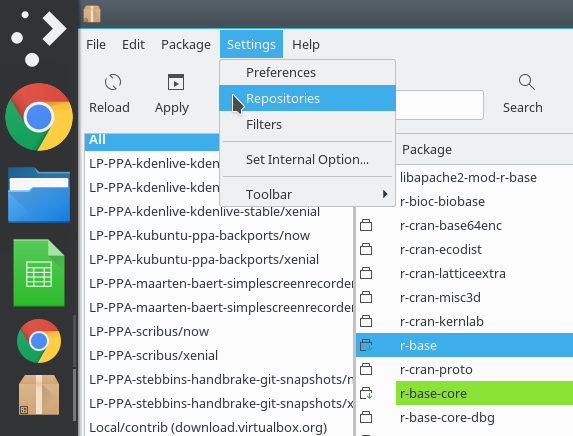In the new Software Sources window, click on “Additional repositories” and you’ll get this window: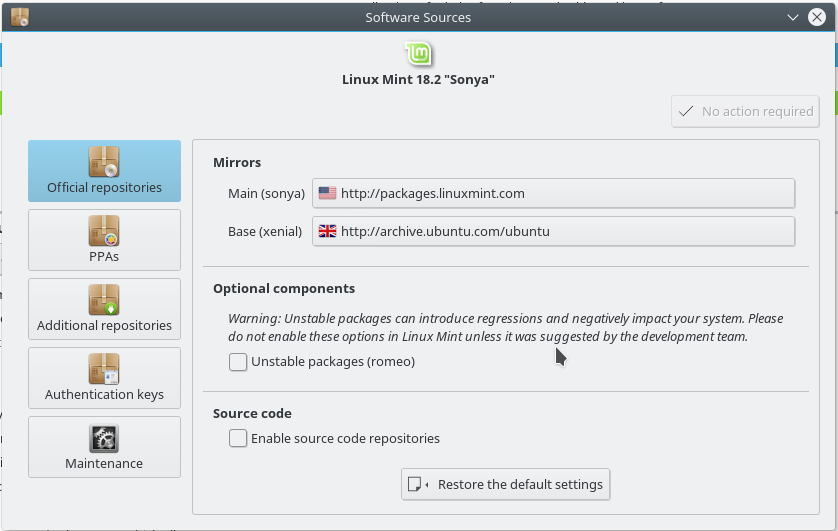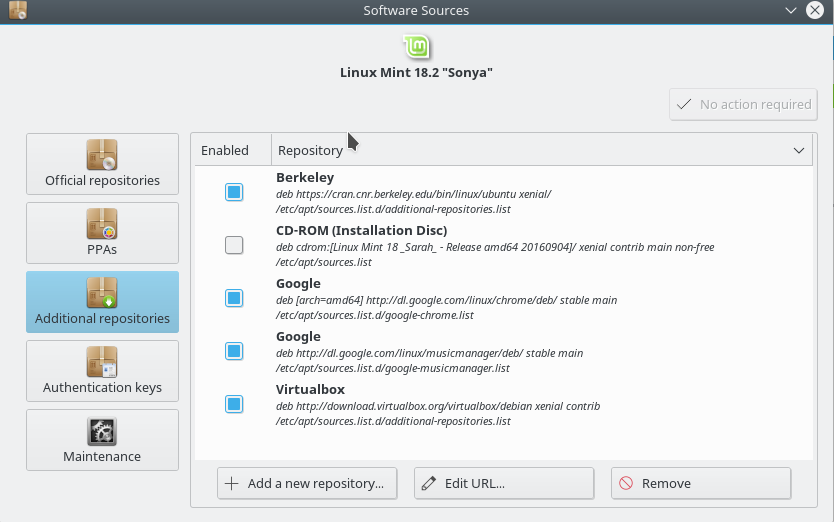Click on Add a new repository. You’ll get this window: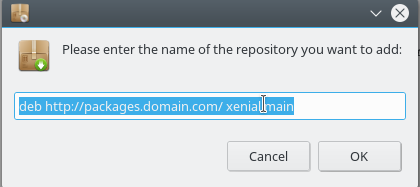The exact information you put into that window will vary based on which mirror you chose. Here is what I added in mine:

deb xenial/

In order to ensure you have the right files and to follow best security practices, you should install the signing key as well. Directions for installing the signing key are found here, but it can be done with a simple command from a terminal:

sudo apt-key adv –keyserver keyserver.ubuntu.com –recv-keys E084DAB9

Once you have done all of that, you can install R from Synaptic.

First, open Synaptic, which will require your password. You’ll get the basic Synaptic Package Manager window: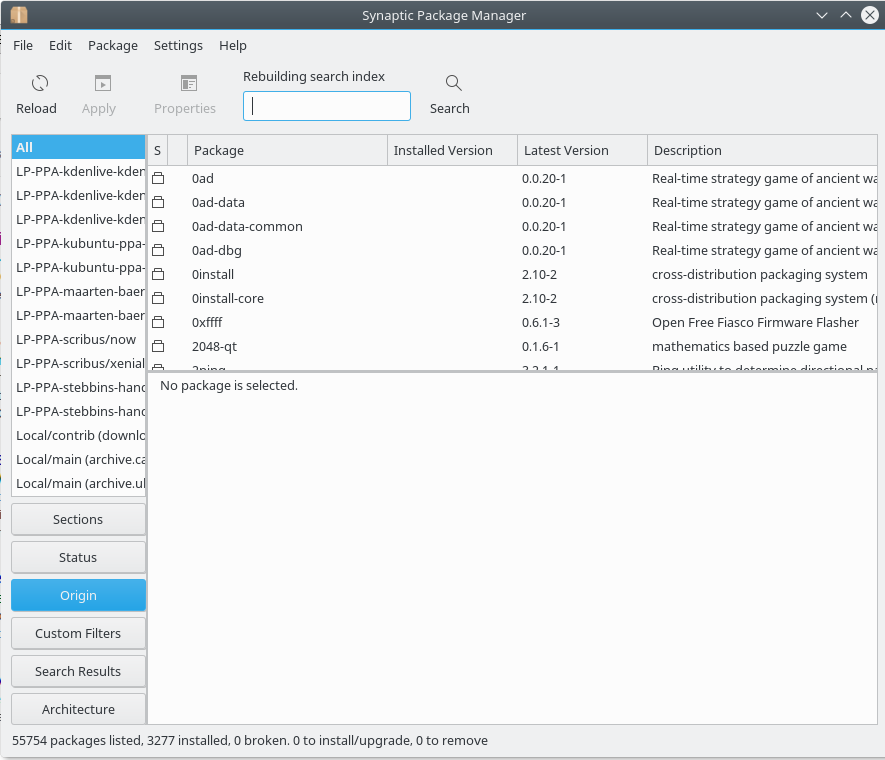Next, in the search box, search for “r-base”. Right-click it and select “Mark for installation” to install “r-base”: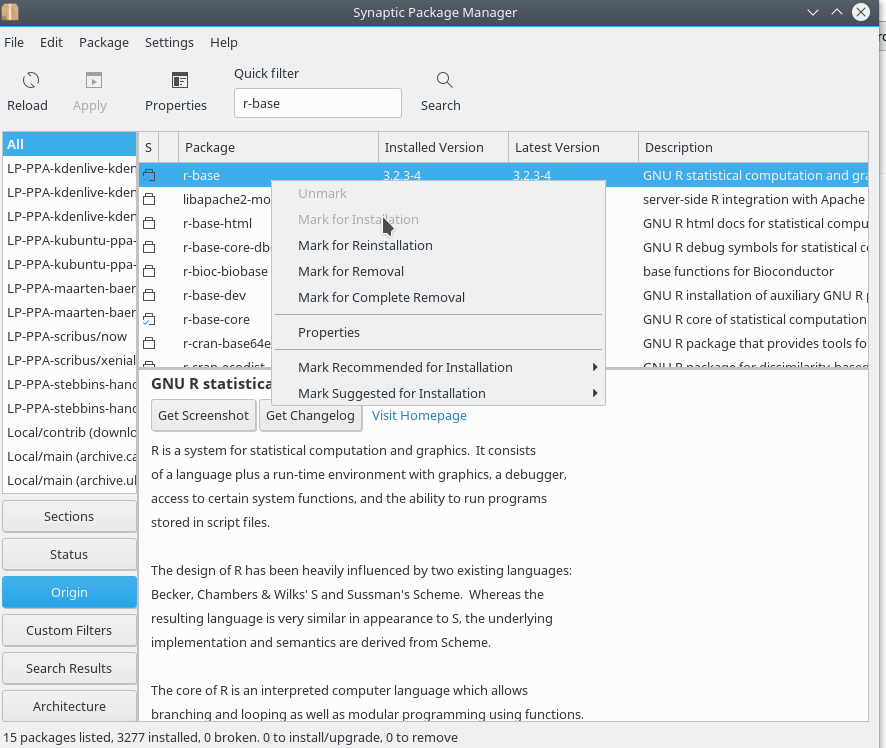In the above screenshot, I have already installed r-base, so the option “Mark for installation” is greyed out. But, obviously, that’s what I already did. When you select this, Synaptic will automatically select all the other necessary packages (there are about 10 to 15 additional packages necessary for R to run: r-cran-class, r-cran-lattice, r-cran-spatial, r-cran-survival, r-cran-codetools, r-cran-nnet, r-cran-mass, r-cran-boot, r-cran-nlme, r-cran-rpart, r-cran-cluster, r-cran-kernsmooth, r-cran-foreign, r-cran-mgcv, r-cran-matrix, r-recommended, r-base-core).

If you plan on installing any other R packages, it’s not a bad idea to also install “r-base-dev,” as it helps fill in dependencies for other packages.

Once you’ve selected r-base, hit Apply in Synaptic and all the software will be installed.

You now have the base software for R installed.

To open the R environment in a terminal, launch a terminal and simply type “R” at the prompt, like this: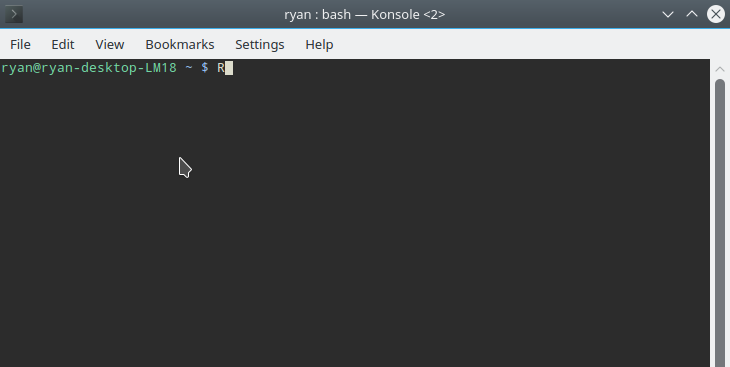Here’s where things can get a little complicated. To do different things in R requires various libraries or packages. Some of these can be installed using the R terminal while others need to be installed from your Linux distribution’s repositories. To install a library or package using the R terminal, you use the following command once you have opened the R environment:

install.packages(“PACKAGENAME”)

The first time you run this, the R environment will ask you to select a mirror.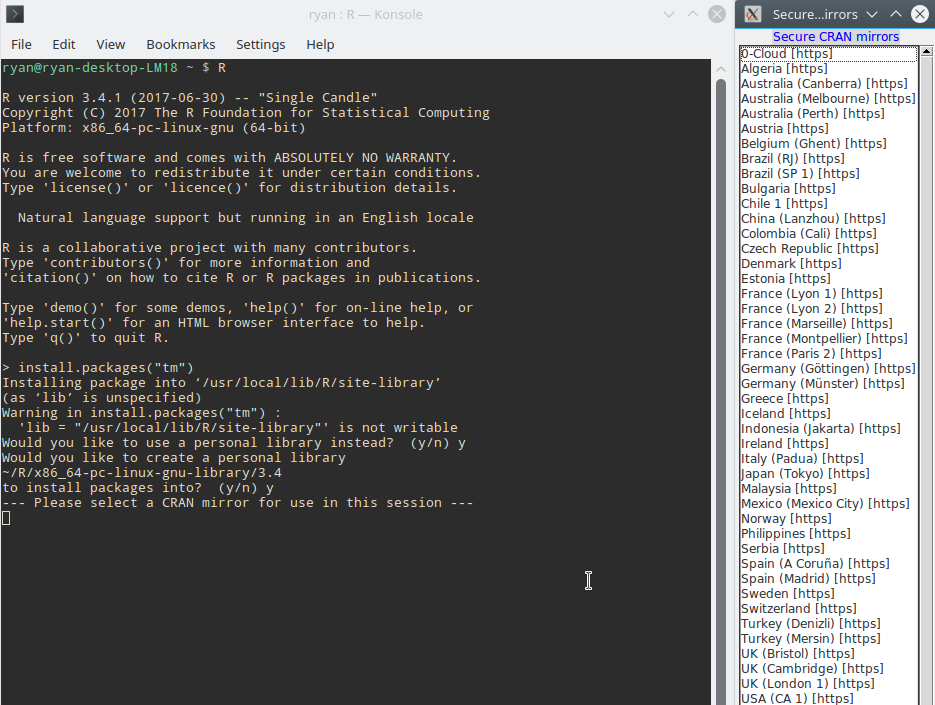Choose one close to your location. R will then install the package, assuming you type everything correctly.

NOTES:

Before you start trying to install additional R packages, it’s a very good idea to install the following Linux packages:

r-base-dev build-essential

If you run into an error message, there are several possibilities. First, check to make sure you typed everything correctly. R is not forgiving on spelling mistakes. Second, if the error is something like:

installation of package ‘PACKAGENAME’ had non-zero exit status

Or

dependency ‘PACKAGENAME’ is not available

There is a good chance that you need to install a package or library using Synaptic (or from a terminal using apt). For instance, to install the “tm” package, there is an unsatisfied dependency (meaning, a library or package that needs to be installed but cannot be installed using the R installer). The dependency is the ‘slam’ package. This can be installed using Synaptic (or, from a terminal, using the command “sudo apt-get install r-cran-slam”). Once you’ve installed the dependency, try re-installing the package and the error messages should go away.

NOTES:

I also have found that I like RStudio as an IDE for working with R. It’s a little bit friendlier to use than a straight command line interface as it keeps track of variables and loaded libraries. The personal version for your desktop can be downloaded here.

And a note on RStudio on Linux. I regularly get an offset from the cursor location and where the cursor actually is in the command window. It turns out this is a font issue. If you go up to Tools -> Global Options -> Appearance and change the font to anything else, this problem will go away.

398 total views